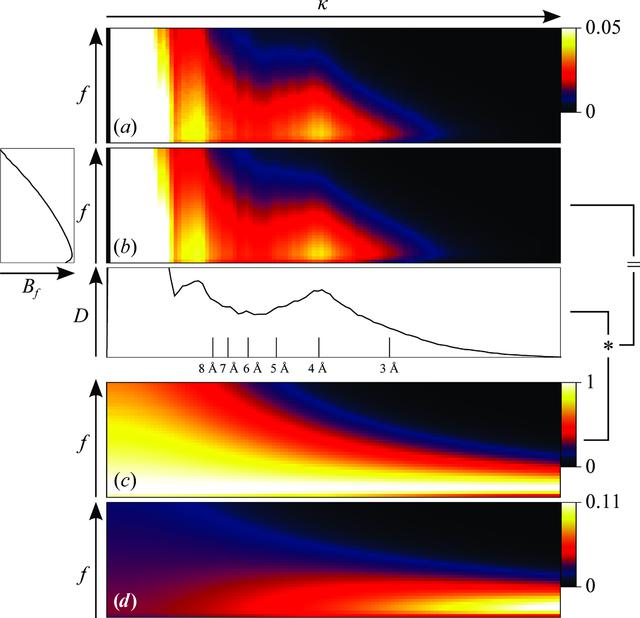disable zoom     view article Figure 2 An illustration of FCC-based B-factor fitting using the β-galactosidase data set as an example (best viewed in colour). (a) The FCC computed using (20)as a function of spatial frequency κ and frame f. (b) A fit of Bf, Cf and Dκ according to (21)with plots of Bf and Dκ shown in relation. (c) The same fit with all Dκ set to 1 (i.e. the numerator of equation 22). (d) The normalized weights wκ,f as given by (22). The asterisk indicates a multiplication.

IUCrJ
ISSN: 2052-2525